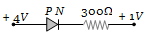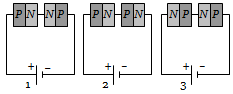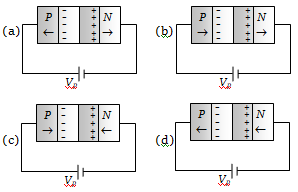PN-junction diode works as a insulator, if connected

(a) To A.C.                              (b) In forward bias
(c) In reverse bias                   (d) None of these

Concept Questions :-

Applications of PN junction
High Yielding Test Series + Question Bank - NEET 2020

Difficulty Level:

The reverse biasing in a PN junction diode

1. Decreases the potential barrier

2. Increases the potential barrier

3. Increases the number of minority charge carriers

4. Increases the number of majority charge carriers

Concept Questions :-

PN junction
High Yielding Test Series + Question Bank - NEET 2020

Difficulty Level:

The electrical resistance of depletion layer is large because

1. It has no charge carriers

2. It has a large number of charge carriers

3. It contains electrons as charge carriers

4. It has holes as charge carriers

Concept Questions :-

Types of semiconductors
High Yielding Test Series + Question Bank - NEET 2020

Difficulty Level:

In the circuit given below, the value of the current is(a) 0 amp                          (b) ${10}^{-2}$ amp
(c) ${10}^{2}$ amp                       (d) ${10}^{-3}$amp

Concept Questions :-

Applications of PN junction
High Yielding Test Series + Question Bank - NEET 2020

Difficulty Level:

The PN junction diode is used as

(a) An amplifier                       (b) A rectifier
(c) An oscillator                       (d) A modulator

Concept Questions :-

Applications of PN junction
High Yielding Test Series + Question Bank - NEET 2020

Difficulty Level:

Two PN-junctions can be connected in series by three different methods as shown in the figure. If the potential difference in the junctions is the same, then the correct connections will be(a) In the circuit (1) and (2)                    (b) In the circuit (2) and (3)
(c) In the circuit (1) and (3)                    (d) Only in the circuit (1)

Concept Questions :-

PN junction
High Yielding Test Series + Question Bank - NEET 2020

Difficulty Level:

In the depletion region of an unbiased P-N junction diode there are

(a) Only electrons

(b) Only holes

(c) Both electrons and holes

(d) Only fixed ions

Concept Questions :-

PN junction
High Yielding Test Series + Question Bank - NEET 2020

Difficulty Level:

On increasing the reverse bias to a large value in a PN-junction diode, current

(a) Increases slowly                  (b) Remains fixed
(c) Suddenly increases              (d) Decreases slowly

Concept Questions :-

PN junction
High Yielding Test Series + Question Bank - NEET 2020

Difficulty Level:

In the case of forward biasing of PN-junction, which one of the following figures correctly depicts the direction of flow of carriersConcept Questions :-

PN junction
High Yielding Test Series + Question Bank - NEET 2020

Difficulty Level:

A semiconductor device is connected in a series circuit with a battery and a resistance. A current is found to pass through the circuit. If the polarity of the battery is reversed, the current drops almost to zero. The device may be

(a) A P-type semiconductor                (b) An N-type semiconductor
(c) A PN-junction                               (d) An intrinsic semiconductor

Concept Questions :-

PN junction